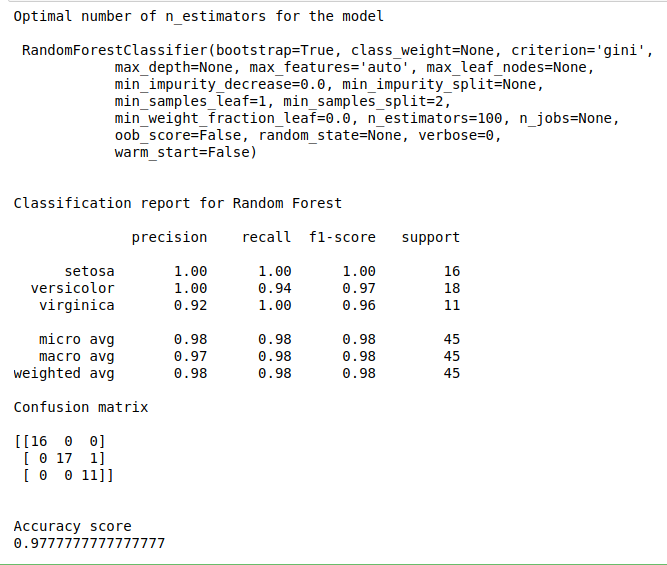• #5, First Floor, 4th Street Dr. Subbarayan Nagar Kodambakkam, Chennai-600 024 Landmark : Samiyar Madam
• pro@slogix.in
• +91- 81240 01111

### How to find optimal number of n_estimators in Random forest algorithm in python?

###### Description

To find best fit n_estimators in random forest algorithm to improve performance of the model

Iris data set

#### Output

Improved classification results.

###### Process

Import the library.

Split the data train and test.

Define the number of n_estimators.

Fit the train data into Gridsearch model.

Find the optimal number of n_estimators.

Improve the model performance.

Calculate precision, recall and accuracy.

###### Sample Code

#import libraries
import warnings
warnings.filterwarnings(“ignore”)
import numpy as np
import pandas as pd
from sklearn.model_selection import train_test_split
from sklearn.ensemble import RandomForestClassifier
from sklearn.model_selection import GridSearchCV
from sklearn.metrics import classification_report, confusion_matrix, accuracy_score

#load the sample data from iris.csv file

#Make it as a data frame
df = pd.DataFrame(data)

#feature variables
X = df.iloc[:,0:4]
y = df.iloc[:,4]

#Split the data into train and testing
X_train, X_test, y_train, y_test = train_test_split(X, y, test_size=0.3, random_state=0)

#Random forest
rf = RandomForestClassifier()
#create a dictionary of all values we want to test for n_estimators
params_rf = {‘n_estimators’: [50, 100, 200]}
#use gridsearch to test all values for n_estimators
rf_gs = GridSearchCV(rf, params_rf, cv=5)
#fit model to training data
rf_gs.fit(X_train, y_train)
rf_best = rf_gs.best_estimator_
print(“Optimal number of n_estimators for the model\n\n”,rf_best)
y_pred = rf_best.predict(X_test)

#Evaluate the model
print(“\n”)
print(“Classification report for Random Forest\n”)
print(classification_report(y_test, y_pred))
print(“Confusion matrix\n”)
print(confusion_matrix(y_test, y_pred))
print(“\n”)
print(“Accuracy score”)
print(accuracy_score(y_test, y_pred))

###### Screenshots